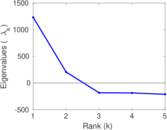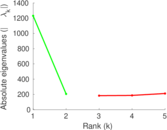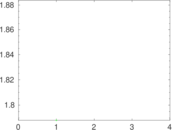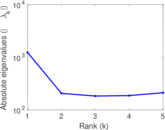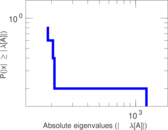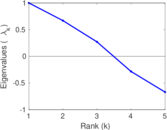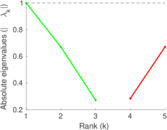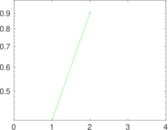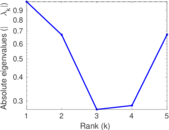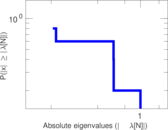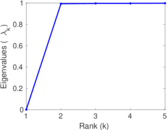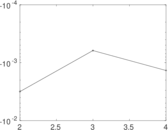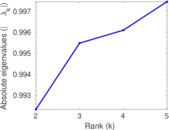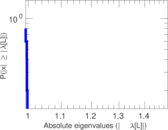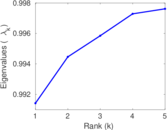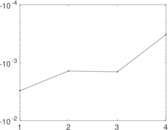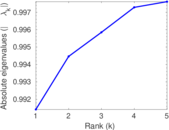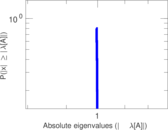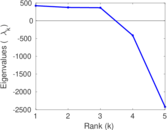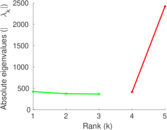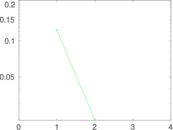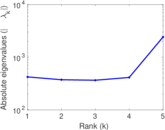# Haggle

This undirected network represents contacts between people measured by carried wireless devices. A node represents a person; an edge between two persons shows that there was a contact between them.

 Code `HA` Internal name `contact` Name Haggle Data source http://www.cl.cam.ac.uk/research/srg/netos/haggle/ AvailabilityDataset is available for download Consistency checkDataset passed all tests Category Human contact network Node meaning Person Edge meaning Contact Network format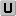Unipartite, undirected Edge typeUnweighted, multiple edges Temporal dataEdges are annotated with timestamps LoopsDoes not contain loops

## Statistics

 Size n = 274 Volume m = 28,244 Unique edge count m̿ = 2,899 Loop count l = 0 Wedge count s = 118,281 Claw count z = 9,250,623 Cross count x = 255,553,498 Triangle count t = 22,332 Square count q = 848,067 4-Tour count T4 = 7,261,908 Maximum degree dmax = 2,092 Average degree d = 206.161 Fill p = 0.077 511 3 Average edge multiplicity m̃ = 9.742 67 Size of LCC N = 274 Diameter δ = 4 50-Percentile effective diameter δ0.5 = 1.949 57 90-Percentile effective diameter δ0.9 = 2.792 77 Median distance δM = 2 Mean distance δm = 2.415 27 Gini coefficient G = 0.841 739 Balanced inequality ratio P = 0.110 360 Relative edge distribution entropy Her = 0.794 985 Power law exponent γ = 1.672 86 Tail power law exponent γt = 1.501 00 Tail power law exponent with p γ3 = 1.501 00 p-value p = 0.000 00 Degree assortativity ρ = −0.474 322 Degree assortativity p-value pρ = 2.635 98 × 10−237 Clustering coefficient c = 0.566 414 Spectral norm α = 1,231.03 Algebraic connectivity a = 0.992 336 Spectral separation |λ1[A] / λ2[A]| = 5.811 49 Non-bipartivity bA = 0.827 927 Normalized non-bipartivity bN = 0.466 542 Algebraic non-bipartivity χ = 0.827 831 Spectral bipartite frustration bK = 0.013 349 0 Controllability C = 192 Relative controllability Cr = 0.700 730

## Plots

### Fruchterman–Reingold graph drawing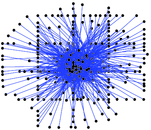### Degree distribution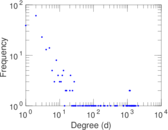### Cumulative degree distribution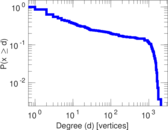### Lorenz curve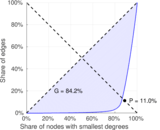### Spectral distribution of the adjacency matrix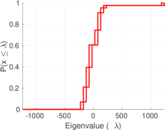### Spectral distribution of the normalized adjacency matrix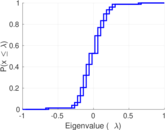### Spectral distribution of the Laplacian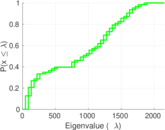### Spectral graph drawing based on the adjacency matrix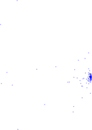### Spectral graph drawing based on the Laplacian### Spectral graph drawing based on the normalized adjacency matrix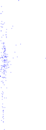### Degree assortativity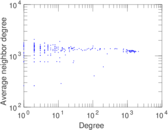### Zipf plot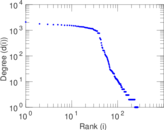### Hop distribution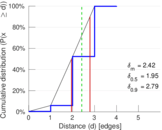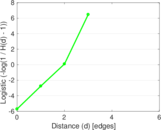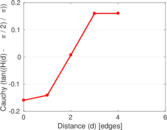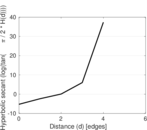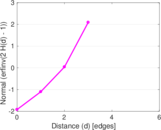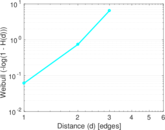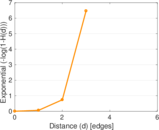### Double Laplacian graph drawing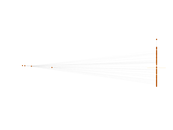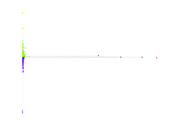### Delaunay graph drawing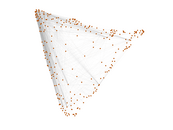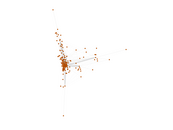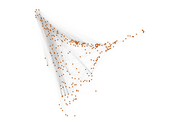### Edge weight/multiplicity distribution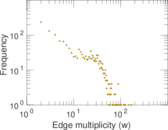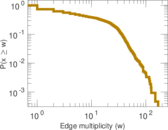### Clustering coefficient distribution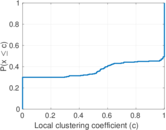### Average neighbor degree distribution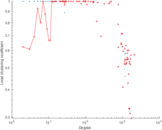### Temporal distribution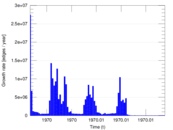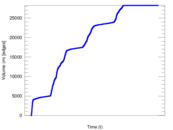### Temporal hop distribution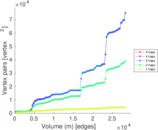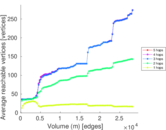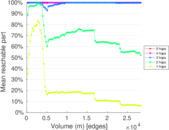### Diameter/density evolution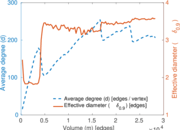### Inter-event distribution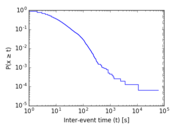### Node-level inter-event distribution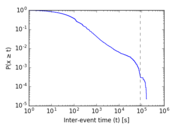### Matrix decompositions plots